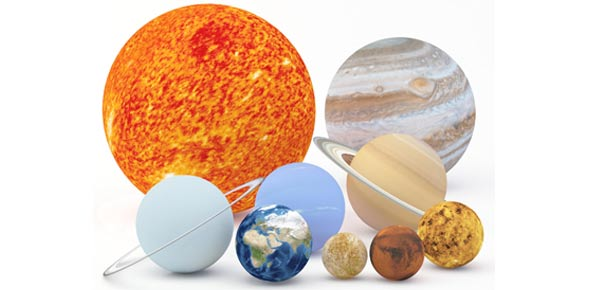# Astronomy Exam 2 Part 2

10 Questions | Attempts: 148
ShareSettingsThere’s so much to learn about the universe because it continues to change and has very mysterious things. For example there is a mysterious form of matter that cannot be detected by normal means. The Astron 100 class gives you a lot of information about our universe. Test out what you know by taking the test below.

• 1.
Which of the following nuclear fuels does an one solar mass star use over the course of its entire evolution?
• A.

A. hydrogen and helium

• B.

B. hydrogen

• C.

C. hydrogen, helium and carbon

• D.

D. hydrogen, helium, carbon, and neon

• E.

E. hydrogen, helium, carbon, neon, and oxygen

• 2.
The most important characteristic of a star for determining its lifetime is its
• A.

A. temperature

• B.

B. color

• C.

C. distance from the main sequence

• D.

• E.

E. mass

• 3.
Which element from the following list does not liberate energy when ”burned” via nuclear fusion in the center of a star?
• A.

A. helium

• B.

B. carbon.

• C.

C. iron.

• D.

D. silicon.

• E.

E. neon.

• 4.
A neutron star is expected to spin rapidly because
• A.

A. it has high orbital velocities.

• B.

B. it has high densities.

• C.

C. it has high temperatures.

• D.

D. it conserved angular momentum when it collapsed.

• E.

E. the energy from the supernova explosion that formed them made them spin faster.

• 5.
Which of the following is true of pulsars?
• A.

A. They have very strong magnetic fields.

• B.

B. They rotate very rapidly.

• C.

C. They are neutron stars.

• D.

D. They have a density approximately equal to the density of an atomic nucleus.

• E.

E. All of the above are true.

• 6.
A white dwarf is supported against collapse by
• A.

A. neutron drip.

• B.

B. neutrino pressure.

• C.

C. electron degeneracy pressure.

• D.

D. neutron degeneracy pressure.

• E.

E. a central black hole.

• 7.
In a supernova explosion
• A.

A. the star may shine as brightly as billions of stars.

• B.

B. elements heavier than iron are formed.

• C.

C. material that later formed the Earth and us humans was distributed between the stars

• D.

D. matter is ejected at tens of thousands of kilometers per second.

• E.

E. All of the above.

• 8.
Which of the following stars is most dense?
• A.

A. a supergiant star

• B.

B. a white dwarf

• C.

C. a main sequence star

• D.

D. a giant star

• E.

E. the sun

• 9.
If two stars have the same spectral type, then they must have approximately the same
• A.

A. mass.

• B.

B. parallactic distance.

• C.

C. surface temperature.

• D.

D. chemical composition.

• E.

E. luminosity.

• 10.
Which of the following can escape from inside the event horizon of a black hole?
• A.

A. particles of matter.

• B.

B. particles of antimatter.

• C.

C. visible light.

• D.

D. X-rays.

• E.

E. None of the above.

## Related TopicsBack to top
×

Wait!
Here's an interesting quiz for you.